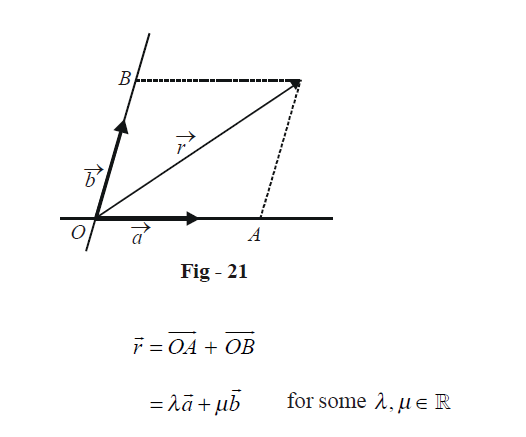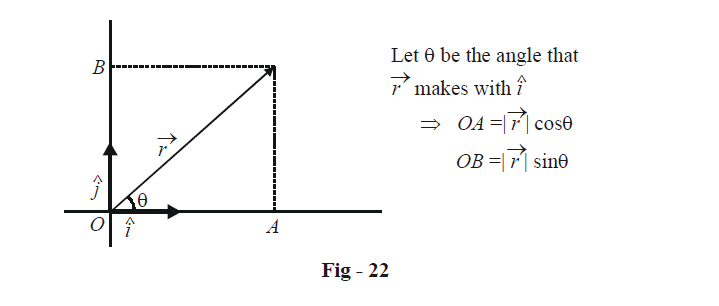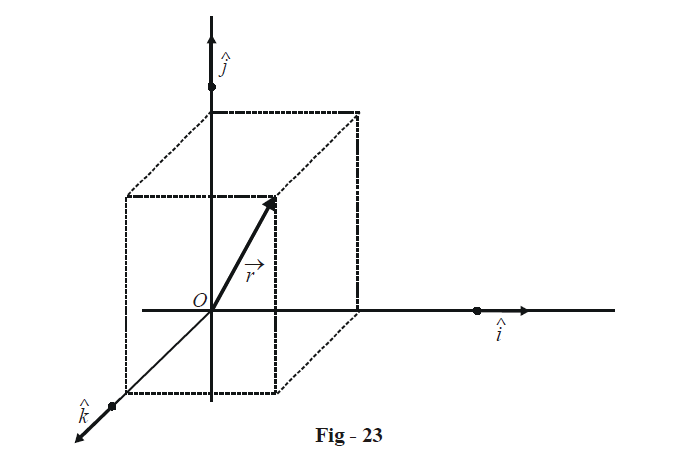# Resolution Of Vectors

RESOLUTION OF A VECTOR IN A GIVEN BASIS

Consider two non-collinear vectors $$\vec a\,\,{\text{and}}\,\,\vec b$$; as discussed earlier, these will form a basis of the plane in which they lie. Any vector  $$\vec r$$ in the plane of $$\vec a\,\,{\text{and}}\,\,\vec b$$ can be expressed as a linear combination of $$\vec a\,\,{\text{and}}\,\,\vec b$$:The vectors $$\overrightarrow {OA} \,\,\,{\text{and}}\,\,\overrightarrow {OB}$$ are called the components of the vector $$\vec r$$ along the basis formed by $$\vec a\,\,{\text{and}}\,\,\vec b$$ . This is also stated by saying that the vector $$\vec r$$ when resolved along the basis formed by $$\vec a\,\,{\text{and}}\,\,\vec b$$ , gives the components  $$\overrightarrow {OA} \,\,\,{\text{and}}\,\,\overrightarrow {OB}$$ . Also, as discussed earlier, the resolution of any vector along a given basis will be unique.

We can extend this to the three dimensional case: an arbitrary vector can be resolved along the basis formed by any three non-coplanar vectors, giving us three corresponding components. Refer to Fig - 20 for a visual picture.

RECTANGULAR  RESOLUTION

Let us select as the basis for a plane, a pair of unit vector $$\hat i\,\,{\text{and}}\,\,\hat j$$ perpendicular to each other.Any vector $$\vec r$$ in this basis can be written as

\begin{align}&\vec r = \overrightarrow {OA} + \overrightarrow {OB} \hfill \\&\;= \left( {\left| {\vec r} \right|\cos \theta } \right)\hat i + \left( {\left| {\vec r} \right|\sin \theta } \right)\hat j \hfill \\&\;= x\hat i + y\hat j \hfill \\ \end{align}

where x and y are referred to as the x and y components of  $$\vec r$$.

For 3-D space, we select three unit vectors $$\hat i,\hat j\,\,{\text{and}}\,\,\hat k$$ each perpendicular to the other two.In this case, any vector $$\vec r$$ will have three corresponding components, generally denoted by x, y and z. We thus have

$\vec r = x\hat i + y\hat j + z\hat k$

The basis ($$\hat i,\hat j$$) for the two dimensional case and ($$\hat i,\hat j,\,\,\hat k$$) for the three-dimensional case are referred to as rectangular basis and are extremely convenient to work with. Unless otherwise stated, we’ll always be using a rectangular basis from now on. Also, we’ll always be implicitly assuming that we’re working in three dimensions since that automatically covers the two dimensional case.

Vectors
grade 11 | Questions Set 1
Vectors
Vectors
grade 11 | Questions Set 2
Vectors